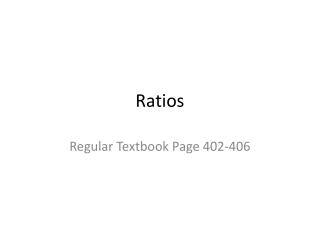DownloadDownload PresentationRatios

# Ratios

Télécharger la présentation## Ratios

- - - - - - - - - - - - - - - - - - - - - - - - - - - E N D - - - - - - - - - - - - - - - - - - - - - - - - - - -
##### Presentation Transcript

1. Ratios Regular Textbook Page 402-406

2. Objective • I can use ratio language to describe the relationship between two quantities.

3. Ratios • What is a ratio? • A ratio is a comparison of two quantities. (Uses division to compare two number) • It shows how many times as great one quantity is to another. • The numbers in the ratio are called terms.

4. There are several ways to write ratios! • There are three ways to write ratios: • Words Numbers • Wins to losses 8 to 3 • Wins8 • losses 3 • Wins:losses 8:3 All three ways are read “ the ratio of blank to blank. So 8:3 is read, “the ratio of eight to three.

5. Write a ratio: A. Keystones wins to losses 7:2 or 7 to 2 or 7/2 Write a ratio of wins to games played for Oberlin. 6 to 10 or 6:10 or 6/10

6. Write me a ratio! • Teacher to students in this room. • Students to teachers. • Boys to girls • Boys to all students.

7. Ty’s Video CollectionComedies=8, Dramas= 3 Cartoons= 2 Sci Fi=1 • Describe the relationship between the drama videos and the Sci Fi videos. • 3 to 1 • Analyze relationships: • The ratio of floor seats to balcony seats in a theater are 20:1. Does the theater have more floor seats or more balcony seats. How do you know?

8. Equivalent Ratios are? • Equivalent Ratios: ratios that name the same comparison or that have the same value.

9. Equivalent Ratios • You can find equivalent ratios by using table or multiplying or dividing both terms of the ratio by the same number.

10. Multiply both terms by the same number. • Ratio: 3 cats to 5 dogs • You can write an equivalent ratio by multiplying both terms by the same number. • So multiply both terms by 2. • An equivalent ratio would be • 6 to 10

11. 5n • 7 35 • N = • What did you do to 7 to get to 35. You must do the same to 5.

12. Some more equivalent Ratios • Write me an equivalent ratio: • 6:21 • 3 9

13. You try! • Write a ratio to triangle to rectangles in three ways. • Write me three equivalent ratio for triangles to rectangles

14. Multi step The ratio of North American Butterflies to south American butterflies at a butterfly park is 5:3. The ratio of South American butterflies to European butterflies is 3:2. There are 30 North American Butterflies at the park. How many South American butterflies are there? How many European Butterflies are there?

15. HomeworkWorkbook Pages 101-102Odds